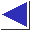# comp.lang.c FAQ list ·Question 16.7

Q: I've got some code that's trying to unpack external structures, but it's crashing with a message about an ``unaligned access.'' What does this mean? The code looks like this:

```struct mystruct {
char c;
long int i32;
int i16;
} s;

char buf, *p;
p = buf;
s.c = *p++;
s.i32 = *(long int *)p;
p += 4;
s.i16 = *(int *)p;
```

A: The problem is that you're playing too fast and loose with your pointers. Some machines require that data values be stored at appropriately aligned addresses. For instance, two-byte short ints might be constrained to sit at even addresses, and four-byte long ints at multiples of four. (See also question 2.12.) By converting a char * (which can point to any byte) to an int * or long int *, and then indirecting on it, you can end up asking the processor to fetch a multibyte value from an unaligned address, which it isn't willing to do.

A better way to unpack external structures is with code like

```	unsigned char *p = buf;

s.c = *p++;

s.i32 = (long)*p++ << 24;
s.i32 |= (long)*p++ << 16;
s.i32 |= (unsigned)(*p++ << 8);
s.i32 |= *p++;

s.i16 = *p++ << 8;
s.i16 |= *p++;
```

This code also gives you control over byte order. (This example, though, assumes that a char is 8 bits, and that the long int and int being unpacked from the ``external structure'' are 32 and 16 bits, respectively.) See question 12.42 (which contains some similar code) for a few explanations and caveats.